Our Discord hit 10K members! 🎉 Meet students and ask top educators your questions.Join Here!

## Educators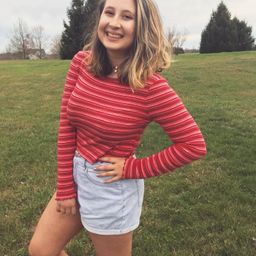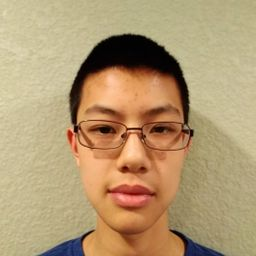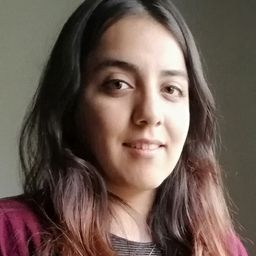### Problem 1

Find the volume of the solid obtained by rotating the region bounded by the given curves about the specified line. Sketch the region, the solid, and a typical disk or washer.
$$y=2-\frac{1}{2} x, y=0, x=1, x=2 ; \text { about the } x-axis$$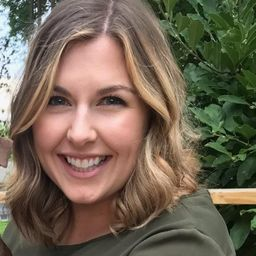Rebecca P.
Numerade Educator

### Problem 2

Find the volume of the solid obtained by rotating the region bounded by the given curves about the specified line. Sketch the region, the solid, and a typical disk or washer.
$$y=1-x^{2}, y=0 ; \quad \text { about the } x-axis$$Rebecca P.
Numerade Educator

### Problem 3

Find the volume of the solid obtained by rotating the region bounded by the given curves about the specified line. Sketch the region, the solid, and a typical disk or washer.
$$x=2 \sqrt{y}, x=0, y=9 ; \quad \text { about the } y-axis$$Rebecca P.
Numerade Educator

### Problem 4

Find the volume of the solid obtained by rotating the region bounded by the given curves about the specified line. Sketch the region, the solid, and a typical disk or washer.
$$y=\ln x, y=1, y=2, x=0 ; \quad \text { about the } y-axis$$Rebecca P.
Numerade Educator

### Problem 5

Find the volume of the solid obtained by rotating the region bounded by the given curves about the specified line. Sketch the region, the solid, and a typical disk or washer.
$$y=x^{3}, y=x, x \geqslant 0 ; \quad \text { about the } x-axis$$Rebecca P.
Numerade Educator

### Problem 6

Find the volume of the solid obtained by rotating the region bounded by the given curves about the specified line. Sketch the region, the solid, and a typical disk or washer.
$$y=\frac{1}{4} x^{2}, y=5-x^{2} ; \quad \text { about the } x-axis$$Rebecca P.
Numerade Educator

### Problem 7

Find the volume of the solid obtained by rotating the region bounded by the given curves about the specified line. Sketch the region, the solid, and a typical disk or washer.
$$y^{2}=x, x=2 y ; \quad \text { about the } y-axis$$Rebecca P.
Numerade Educator

### Problem 8

Find the volume of the solid obtained by rotating the region bounded by the given curves about the specified line. Sketch the region, the solid, and a typical disk or washer.
$$y=\frac{1}{4} x^{2}, x=2, y=0 ; \quad \text { about the } y-axis$$Rebecca P.
Numerade Educator

### Problem 9

Find the volume of the solid obtained by rotating the region bounded by the given curves about the specified line. Sketch the region, the solid, and a typical disk or washer.
$$y=x, y=\sqrt{x} ; \quad \text { about } y=1$$Rebecca P.
Numerade Educator

### Problem 10

Find the volume of the solid obtained by rotating the region bounded by the given curves about the specified line. Sketch the region, the solid, and a typical disk or washer.
$$y=e^{-x}, y=1, x=2 ; \quad \text { about } y=2$$Rebecca P.
Numerade Educator

### Problem 11

Find the volume of the solid obtained by rotating the region bounded by the given curves about the specified line. Sketch the region, the solid, and a typical disk or washer.
$$y=1+\sec x, y=3 ; \quad \text { about } y=1$$

Check back soon!

### Problem 12

Find the volume of the solid obtained by rotating the region bounded by the given curves about the specified line. Sketch the region, the solid, and a typical disk or washer.
$$y=x, y=\sqrt{x} ; \text { about } x=2$$Rebecca P.
Numerade Educator

### Problem 13

The region enclosed by the given curves is rotated about the specified line. Find the volume of the resulting solid.
$$y=1 / x, x=1, x=2, y=0 ; \quad \text { about the } x-axis$$Rebecca P.
Numerade Educator

### Problem 14

The region enclosed by the given curves is rotated about the specified line. Find the volume of the resulting solid.
$$x=2 y-y^{2}, x=0 ; \quad \text { about the } y-axis$$Rebecca P.
Numerade Educator

### Problem 15

The region enclosed by the given curves is rotated about the specified line. Find the volume of the resulting solid.
$$x-y=1, y=x^{2}-4 x+3 ; \quad \text { about } y=3$$Rebecca P.
Numerade Educator

### Problem 16

The region enclosed by the given curves is rotated about the specified line. Find the volume of the resulting solid.
$$x=y^{2}, x=1 ; \quad \text { about } x=1$$Rebecca P.
Numerade Educator

### Problem 17

The region enclosed by the given curves is rotated about the specified line. Find the volume of the resulting solid.
$$y=x^{3}, y=\sqrt{x} ; \quad \text { about } x=1$$Rebecca P.
Numerade Educator

### Problem 18

The region enclosed by the given curves is rotated about the specified line. Find the volume of the resulting solid.
$$y=x^{3}, y=\sqrt{x} ; \quad \text { about } y=1$$Rebecca P.
Numerade Educator

### Problem 19

Set up an integral for the volume of the solid obtained by rotating the region bounded by the given curves about the specified line. Then use your calculator to evaluate the integral correct to five decimal places.
$$y=e^{-x^{2}}, y=0, x=-1, x=1$$
(a) About the $x$ -axis (b) About $y=-1$

Check back soon!

### Problem 20

Set up an integral for the volume of the solid obtained by rotating the region bounded by the given curves about the specified line. Then use your calculator to evaluate the integral correct to five decimal places.
$$y=0, y=\cos ^{2} x,-\pi / 2 \leqslant x \leqslant \pi / 2$$
(a) About the $x$ -axis (b) About y $=1$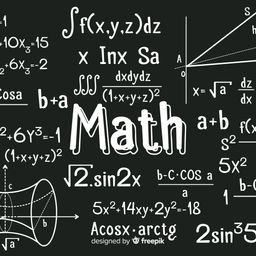Victoria D.
Numerade Educator

### Problem 21

Set up an integral for the volume of the solid obtained by rotating the region bounded by the given curves about the specified line. Then use your calculator to evaluate the integral correct to five decimal places.
$$x^{2}+4 y^{2}=4$$
(a) About $y=2$ (b) About $x=2$

Check back soon!

### Problem 22

Set up an integral for the volume of the solid obtained by rotating the region bounded by the given curves about the specified line. Then use your calculator to evaluate the integral correct to five decimal places.
$$y=x^{2}, x^{2}+y^{2}=1, y \geqslant 0$$
(a) About the $x$ -axis (b) About the $y$ -axisRebecca P.
Numerade Educator

### Problem 23

Use a graph to find approximate $x$ -coordinates of the points of intersection of the given curves. Then use your calculator to find (approximately) the volume of the solid obtained
by rotating about the $x$ -axis the region bounded by these curves.
$$y=2+x^{2} \cos x, \quad y=x^{4}+x+1$$Victoria D.
Numerade Educator

### Problem 24

Use a graph to find approximate $x$ -coordinates of the points of intersection of the given curves. Then use your calculator to find (approximately) the volume of the solid obtained
by rotating about the $x$ -axis the region bounded by these curves.
$$y=3 \sin \left(x^{2}\right), \quad y=e^{x / 2}+e^{-2 x}$$Victoria D.
Numerade Educator

### Problem 25

Use a computer algebra system to find the exact volume of the solid obtained by rotating the region bounded by the given curves about the specified line.
$$y=\sin ^{2} x, y=0,0 \leqslant x \leqslant \pi ; \quad \text { about } y=-1$$Victoria D.
Numerade Educator

### Problem 26

Use a computer algebra system to find the exact volume of the solid obtained by rotating the region bounded by the given curves about the specified line.
$$y=x, y=x e^{1-x / 2} ; \quad \text { about } y=3$$

Check back soon!

### Problem 27

Each integral represents the volume of a solid. Describe the solid.
$$\text { (a) }\pi \int_{0}^{\pi / 2} \cos ^{2} x d x \quad \text { (b) } \pi \int_{0}^{1}\left(y^{4}-y^{8}\right) d y$$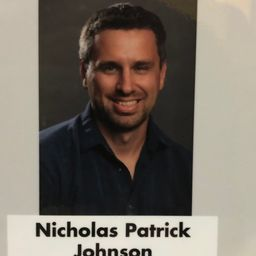Nick J.
Numerade Educator

### Problem 28

Each integral represents the volume of a solid.
Describe the solid.
$$\text { (a) }\pi \int_{2}^{5} y d y \quad \text { (b) } \pi \int_{0}^{\pi / 2}\left[(1+\cos x)^{2}-1^{2}\right] d x$$Victoria D.
Numerade Educator

### Problem 29

A CAT scan produces equally spaced cross-sectional views
of a human organ that provide information about the organ
otherwise obtained only by surgery. Suppose that a CAT
scan of a human liver shows cross-scctions spaced 1.5 $\mathrm{cm}$
apart. The liver is 15 $\mathrm{cm}$ long and the cross-sectional areas,
in square centimeters, are $0,18,58,79,94,106,117,128,$
$63,39,$ and $0 .$ Use the Midpoint Rulc to estimate the volume of the liver.Rebecca P.
Numerade Educator

### Problem 30

A log 10 $\mathrm{m}$ long is cut at 1 -meter intervals and its cross-
sectional areas $A$ (at a distance $x$ from the end of the log)
are listed in the table. Use the Midpoint Rule with $n=5$
to estimate the volume of the log.

Check back soon!

### Problem 31

Find the volume of the described solid $S .$
A right circular cone with height $h$ and base radius $r$Rebecca P.
Numerade Educator

### Problem 32

Find the volume of the described solid $S .$
A frustum of a right circular cone with height $h$ , lower
base radius $R,$ and top radius $r$

Check back soon!

### Problem 33

Find the volume of the described solid $S .$
A cap of a sphere with radius $r$ and height $h$

Check back soon!

### Problem 34

Find the volume of the described solid $S .$
A frustum of a pyramid with square base of side $b,$ square
top of side $a,$ and height $h$
What happens if $a=b ?$ What happens if $a=0 ?$

Check back soon!

### Problem 35

Find the volume of the described solid $S .$
A pyramid with height $h$ and rectangular base with dimensions $b$ and 2$b$Rebecca P.
Numerade Educator

### Problem 36

Find the volume of the described solid $S .$
A pyramid with height $h$ and base an cquilateral triangle
with side $a($ a tetrahedron $)$

Check back soon!

### Problem 37

Find the volume of the described solid $S .$
A tetrahedron with three mutually perpendicular faces and
three mutually perpendicular cdges with lengths 3 $\mathrm{cm}$ ,
$4 \mathrm{cm},$ and 5 $\mathrm{cm}$

Check back soon!

### Problem 38

Find the volume of the described solid $S .$
The base of $S$ is a circular disk with radius $r .$ Parallel cross-sections perpendicular to the base are squares.

Check back soon!

### Problem 39

Find the volume of the described solid $S .$
The base of $S$ is an elliptical region with boundary curve
$9 x^{2}+4 y^{2}=36 .$ Cross-sections perpendicular to the $x$ -axis
are isosceles right triangles with hypotenuse in the base.

Check back soon!

### Problem 40

Find the volume of the described solid $S .$
The base of $S$ is the triangular region with vertices $(0,0),$
$(1,0),$ and $(0,1)$ . Cross-sections perpendicular to the $y$ -axis
are equilateral triangles.

Check back soon!

### Problem 41

Find the volume of the described solid $S .$
The base of $S$ is the same base as in Exercise 40 , but cross-sections perpendicular to the $x$ -axis are squares.Nick J.
Numerade Educator

### Problem 42

Find the volume of the described solid $S .$
The base of $S$ is the region enclosed by the parabola
$y=1-x^{2}$ and the $x$ -axis. Cross-sections perpendicular to
the $y$ -axis are squares.

Check back soon!

### Problem 43

Find the volume of the described solid $S .$
The base of $S$ is the same base as in Exercise $42,$ but cross-sections perpendicular to the $x$ -axis are isosceles triangles
with height equal to the base.Nick J.
Numerade Educator

### Problem 44

The base of $S$ is a circular disk with radius $r .$ Parallel cross-sections perpendicular to the base are isosceles triangles
with height $h$ and unequal side in the base.
(a) Set up an integral for the volume of $S .$
(b) By interpreting the integral as an area, find the volume
of $S .$

Check back soon!

### Problem 45

Some of the pioneers of calculus, such as Kepler and Newton, were inspired by the problem of finding the volumes of wine barrels. (In fact Kepler published a book Stereometria doliorum in 1715 devoted to methods for finding the
volumes of barrels.) They often approximated the shape of
the sides by parabolas.
(a) A barrel with height $h$ and maximum radius $R$ is constructed by rotating about the $x$ -axis the parabola
$y=R-c x^{2},-h / 2 \leqslant x \leqslant h / 2,$ where $c$ is a positive
constant. Show that the radius of each end of the barrel
is $r=R-d,$ where $d=c h^{2} / 4$
(b) Show that the volume enclosed by the barrel is
$$V=\frac{1}{3} \pi h\left(2 R^{2}+r^{2}-\frac{2}{5} d^{2}\right)$$Victoria D.
Numerade Educator

### Problem 46

(a) A model for the shape of a bird's egg is obtained by
rotating about the $x$ -axis the region under the graph of
$$f(x)=\left(a x^{3}+b x^{2}+c x+d\right) \sqrt{1-x^{2}}$$
Use a CAS to find the volume of such an egg.
(b) For a Red-throated Loon, $a=-0.06, b=0.04$
$c=0.1,$ and $d=0.54 .$ Graph $f$ and find the volume
of an egg of this bird.

Check back soon!

### Problem 47

(a) Set up an integral for the volume of a solid torus (the
donut-shaped solid shown in the figure) with radii $r$
and $R .$
(b) By interpreting the integral as an area, find the volume
of the torus.

Check back soon!

### Problem 48

A wedge is cut out of a circular cylinder of radius 4 by two
planes. One plane is perpendicular to the axis of the cylinder. The other intersects the first at an angle of $30^{\circ}$ along a
diameter of the cylinder. Find the volume of the wedge.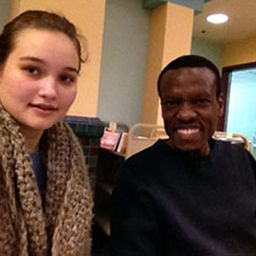Paul A.
California State Polytechnic University, Pomona

### Problem 49

(a) Cavalieri's Principle states that if a family of parallel
planes gives equal cross-sectional areas for two solids
$S_{1}$ and $S_{2},$ then the volumes of $S_{1}$ and $S_{2}$ are equal.
Prove this principle.
(b) Use Cavalieri's Principle to find the volume of the
oblique cylinder shown in the figure.

Check back soon!

### Problem 50

Find the volume common to two circular cylinders, each
with radius $r,$ if the axes of the cylinders intersect at right
angles.

Check back soon!

### Problem 51

Find the volume common to two spheres, each with radius $r$
if the center of each sphere lies on the surface of the other
sphere.

Check back soon!

### Problem 52

A bowl is shaped like a hemisphere with diameter 30 $\mathrm{cm} . \mathrm{A}$
ball with diameter 10 $\mathrm{cm}$ is placed in the bowl and water is
poured into the bowl to a depth of $h$ centimeters. Find the
volume of water in the bowl.

Check back soon!

### Problem 53

A hole of radius $r$ is bored through a cylinder of radius
$R>r$ at right angles to the axis of the cylinder. Set up, but
do not evaluate, an integral for the volume cut out.

Check back soon!

### Problem 54

A hole of radius $r$ is bored through the center of a sphere of
radius $R > r .$ Find the volume of the remaining portion of
the sphere.

Check back soon!